# Basics of Bar Bending Schedule Formulas | BBS Formulas with Example

Basics of Bar Bending Schedule Formulas, bbs formula, Cutting Length Formula, slab, beam, column, footing, staircase, culvert

3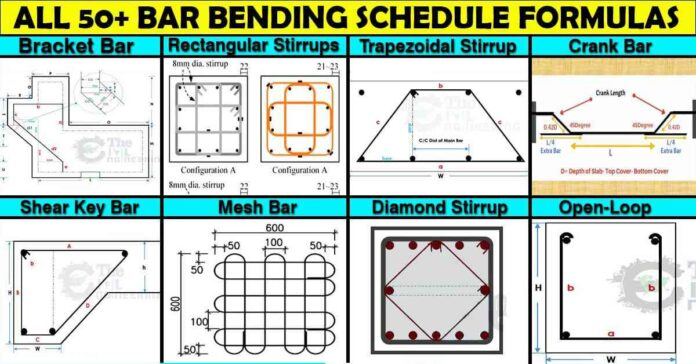# Basics of Bar Bending Schedule Formula | BBS Step by Step Preparation | BBS formulas | Cutting Length Formula | Reinforcement Calculation Excel

In this Article today we will talk about the What is Bar Bending Schedule | Basics of Bar Bending Schedule Formulas | BBS Formulas | Reinforcement Calculation Excel | Cutting Length Formula | How to Make Bar Bending Schedule | Bar Bending Schedule Calculation Formulas

## What is Bar Bending Schedule (BBS) ?

Bar Bending Schedule is a process of Cutting and Bending of Reinforcement bar into required shape. And making it’s list which describes:

• Location & Marking of Bar
• Type of Bar
• Size of Bar
• Cutting Length of Bar
• Number of Bar
• Bending Detail of Bar
• Total Quantity

of each bar in a reinforcement drawing of a structure. This process of listing is called Scheduling.

## Diameter of Reinforcement Bars in MM: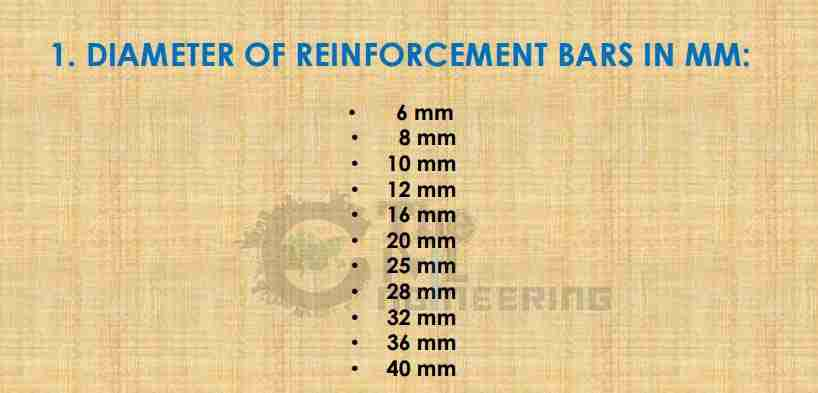## Standard Length of Bar in Meter: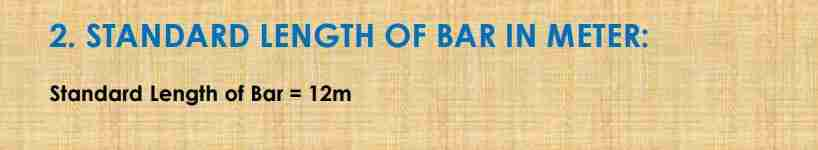## Diameter of Reinforcement Bars in Inches/No’s: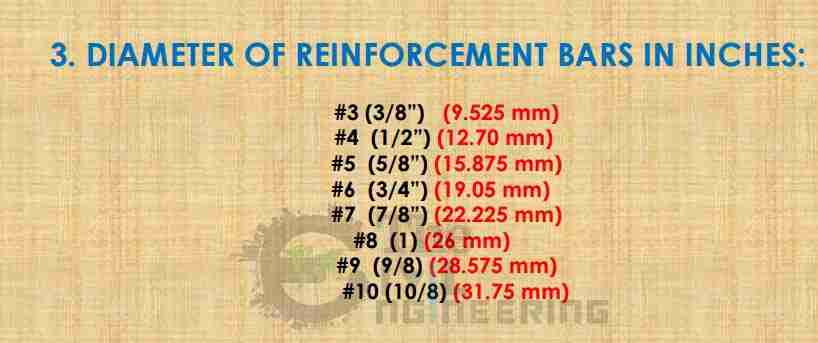## Standard Length of Bar in Feet: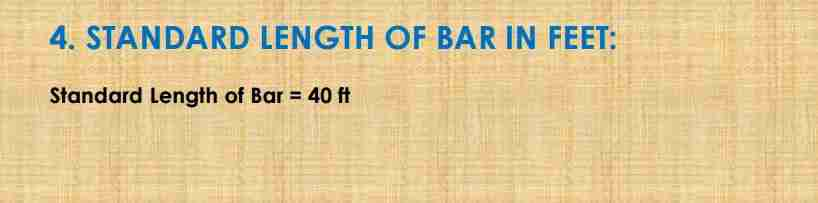## Weight of Bar Per Meter (Kg/m) Formula: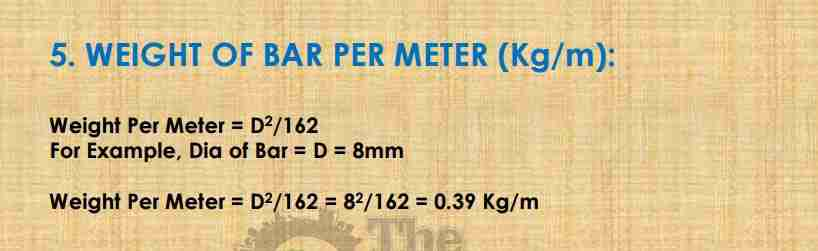Diameter of Bar (mm) Unit weight in kg/m 8 0.395 10 0.617 12 0.889 16 1.580 20 2.469 25 3.858 32 6.321 40 9.877

## Weight of One Bar in Kg/Bar Formula: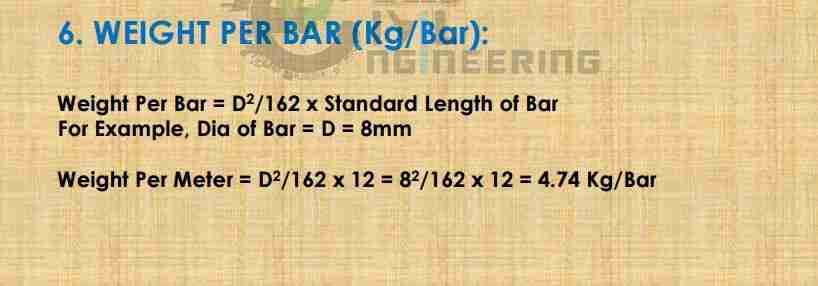## Weight of Bar Per Feet (Kg/ft) Formula: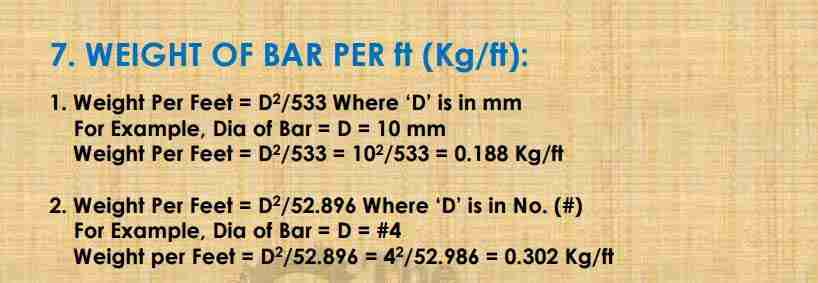## Weight of One Bar in Kg/Bar Formula: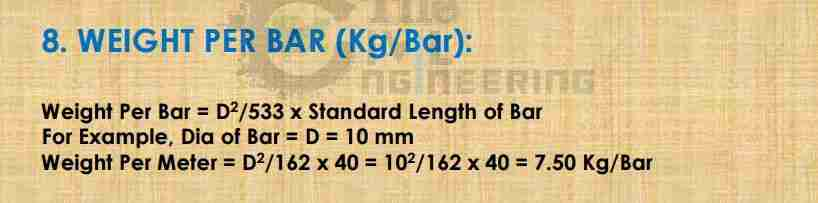## Cutting Length of Main Bar with Hook Formula: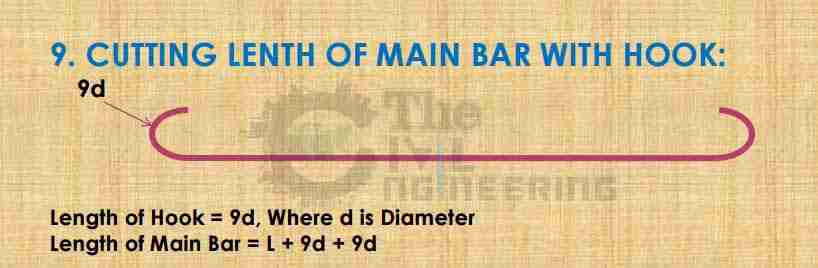Where, L = Span – (Conc. Cover x 2), Note: Hook Length in Stirrups should be 10d or 75mm, where d is dia of bar.

## Cutting Length of Main Bar with Bend Formula: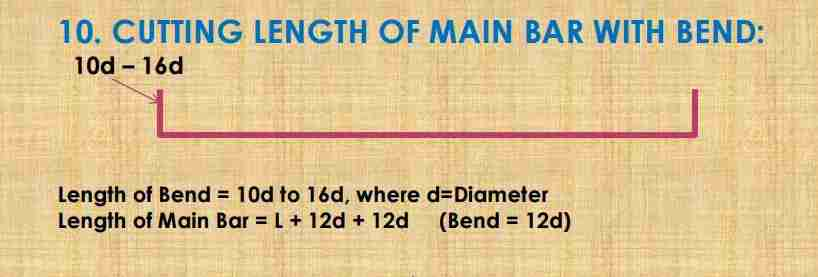Where, L = Span – (Conc. Cover x 2), if you need bend deduction then you should deduct 4d (2d + 2d) for two 90 Deg bends from main bar cutting length.

## Overlapping | Formula :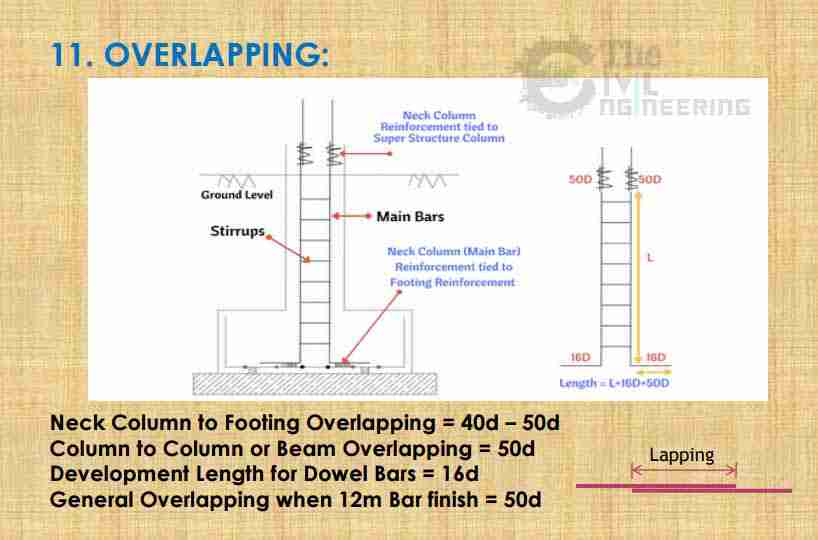## Concrete Cover or Clear Cover in Reinforcement: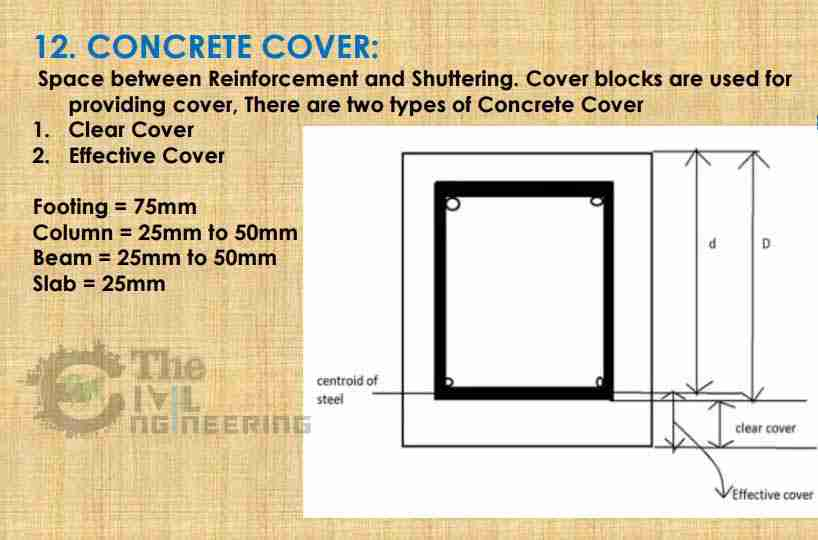Clear Cover for:

• Footing: 50mm
• Column: 40mm
• Beam: 25mm
• Slab: 20mm
• Staircase: 15mm
• Chajja: 15mm
• Pile: 60mm
• Pile Cap: 60mm

## Spacing in Reinforcement: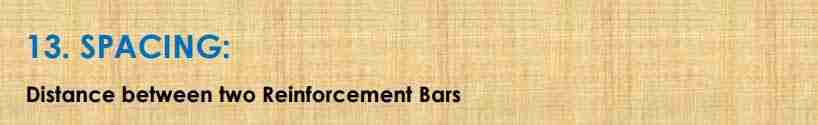## Number of Bars:## Number of Stirrups Formula: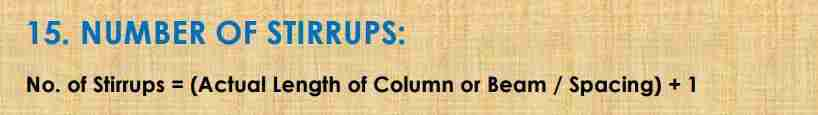## Bend Deduction in Bar Bending Formula:

Bend increases the length of bar. So we need lesser length than we see in the drawing. So cutting length is taken less then the required length:## Cutting Length of Crank or Bent Up Bar Formula: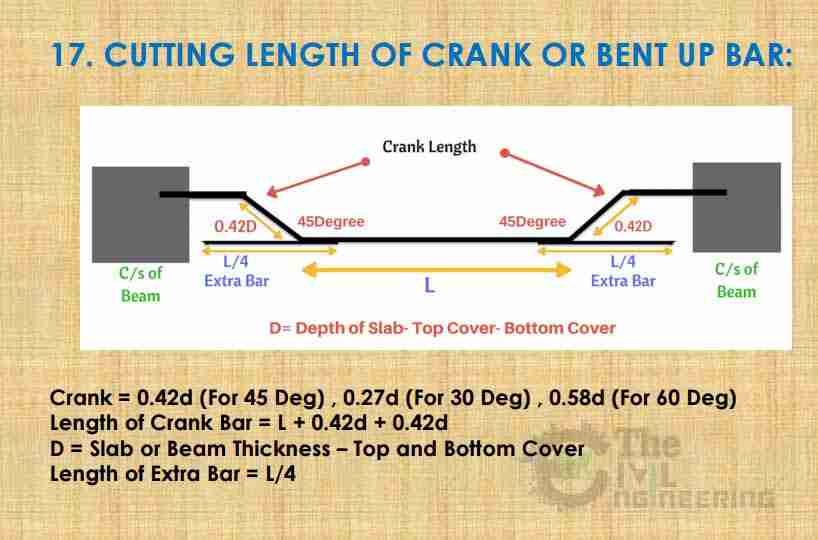Bend Deduction: If you need bend deduction then you should deduct 4d (1d + 1d + 1d + 1d) for four 45 Deg bends from crank or bent up bar cutting length.

## Cutting Length of Rectangular Stirrups Formula: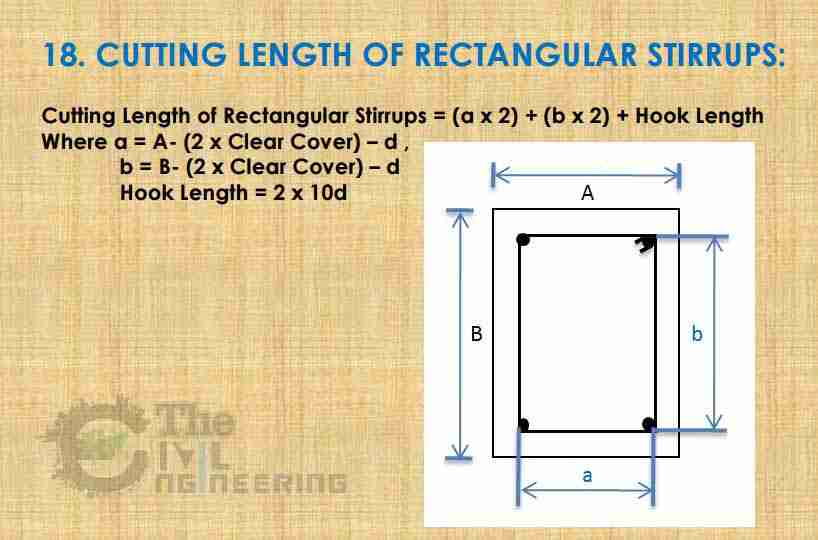Bend Deduction: If you need bend deduction then you should deduct 6d (2d + 2d + 2d) for three 90 Deg bends & 6d (3d + 3d) for two 135 Deg hook bends from rectangular stirrup cutting length.

## Cutting Length of Open-Loop Rectangular Stirrups Formula: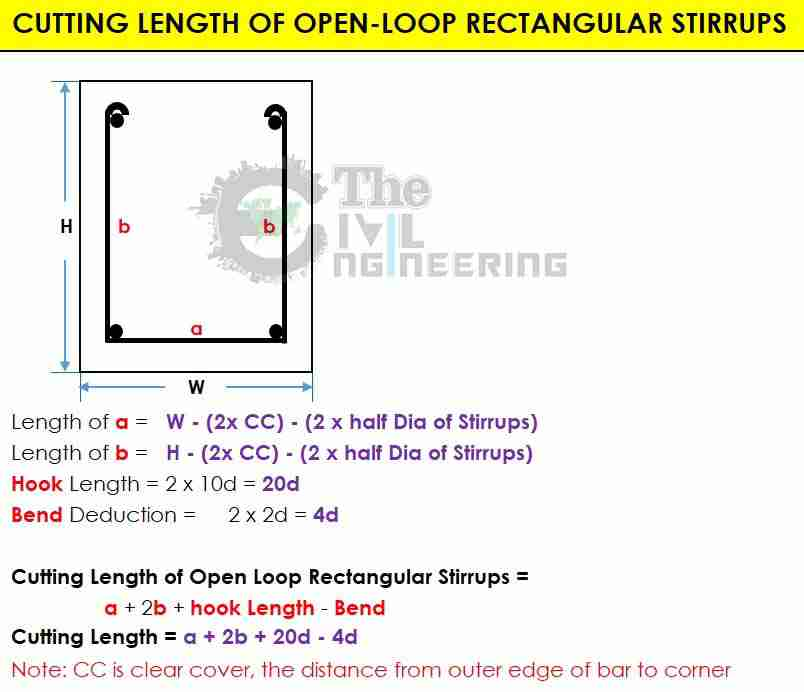## Cutting Length of Triangular Stirrups Formula: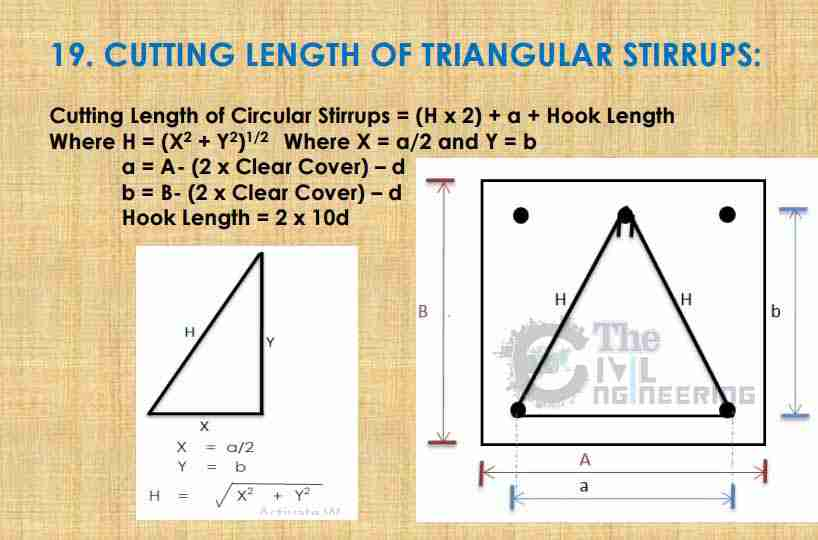Bend Deduction: If you need bend deduction then you should deduct 12d (3d + 3d + 3d + 3d) for four 135 Deg bends from triangular stirrup cutting length.

## Cutting Length of Circular Stirrups Formula: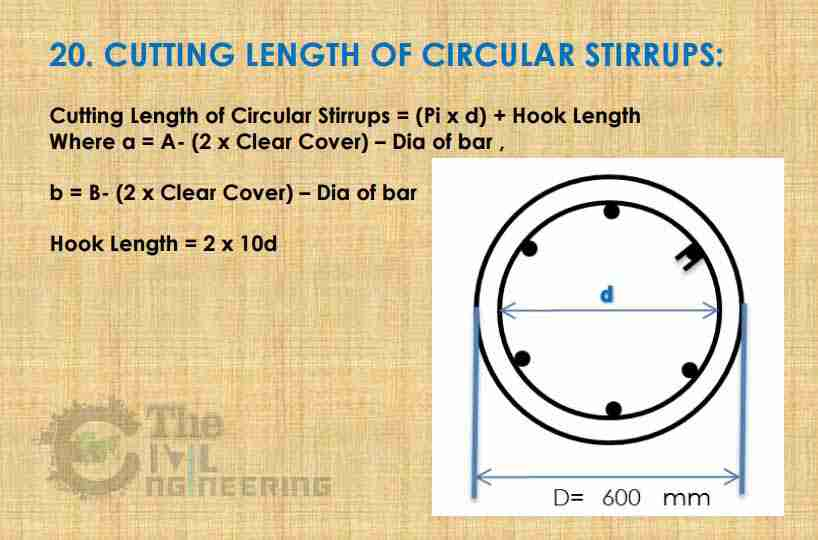Bend Deduction: If you need bend deduction then you should deduct 6d (3d + 3d) for two 135 Deg hook bends from circular stirrup cutting length.

## Cutting Length of Diamond Stirrups Formula: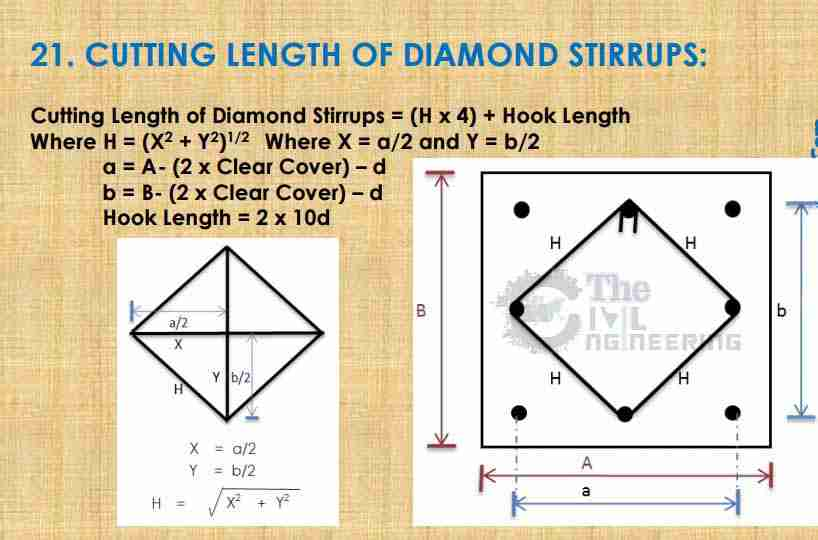Bend Deduction: If you need bend deduction then you should deduct 6d (2d + 2d + 2d) for three 90 Deg bends & 6d (3d + 3d) for two 135 Deg hook bends from diamond stirrup cutting length.

## Cutting Length of Spiral or Helix Stirrups Formula: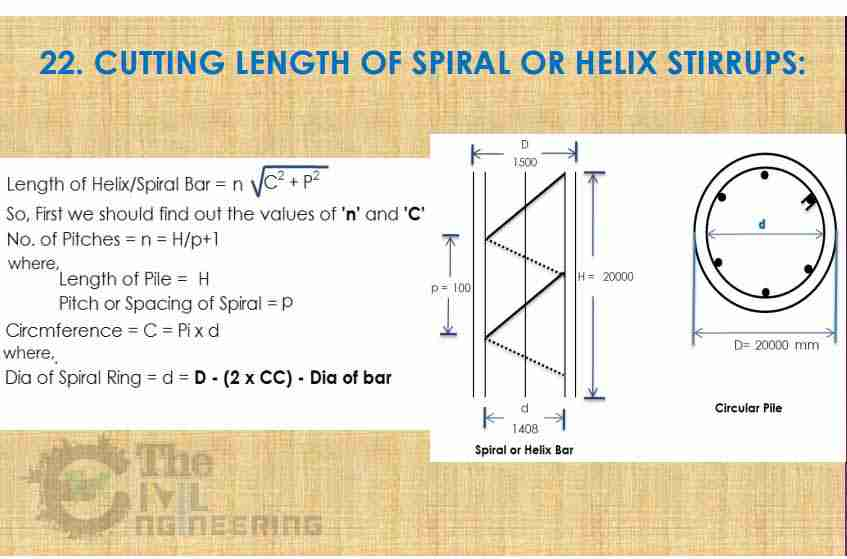Bend Deduction: If you need bend deduction then you should deduct 6d (3d + 3d) for two 135 Deg hook bends from spiral ring cutting length.

## Cutting Length of Chair Bar Formula: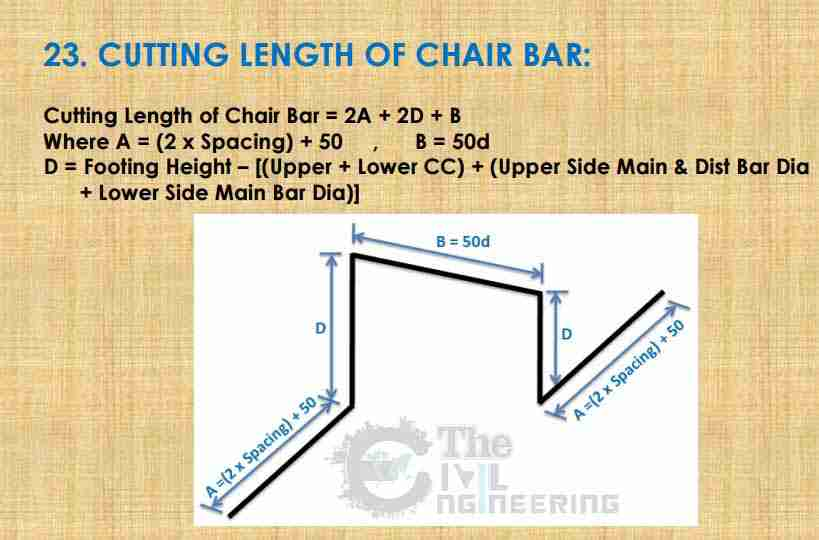Bend Deduction: If you need bend deduction then you should deduct 8d (2d + 2d + 2d) for four 90 Deg bends from chair bar cutting length.

## Cutting Length of Trapezoidal Stirrup Formula: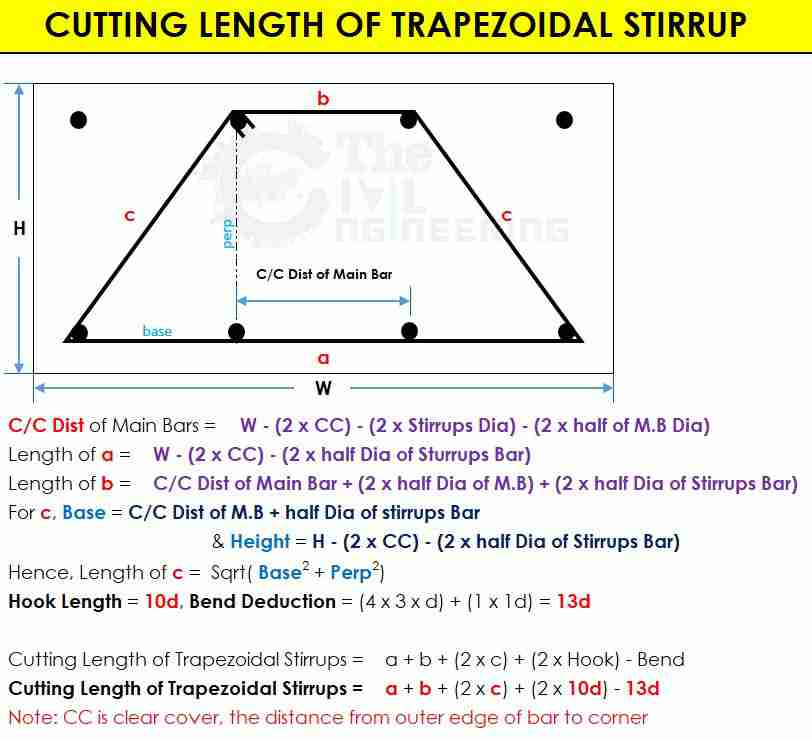## Cutting Length of Shear Key Bar or Stirrup Formula: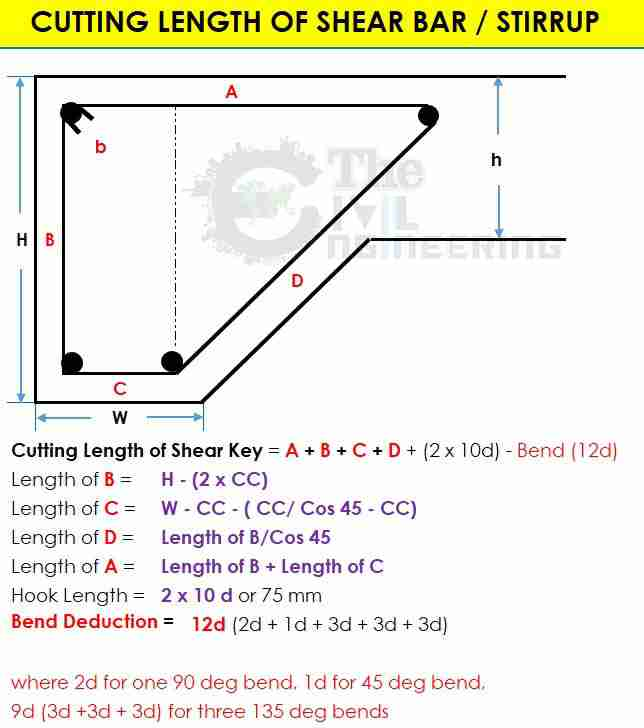## Cutting Length of Haunch Bar Formula: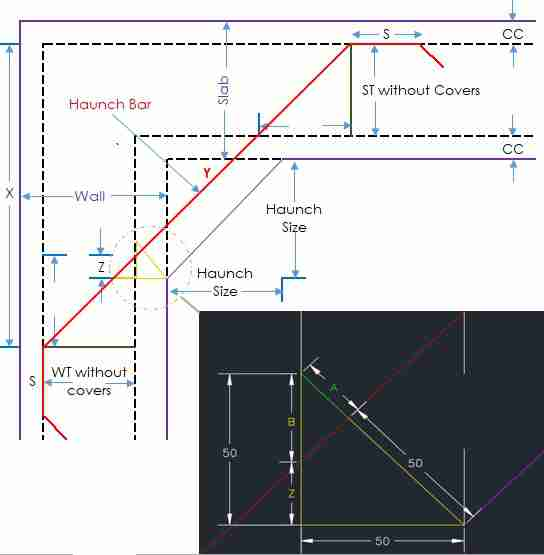Cutting Length of Haunch Bar = S + Y + S (Where S is given as lap)

As we Know that

Y = X / Cos 45

X = (Thickness of wall – Slab Cover) + (Haunch Size) + (Wall Thickness without covers – Z)

Where, Z = Cover – B

B = A / Cos 45 A

we can find the A by using Pythagoras theorem Hyp2 = Base2 + Perp2

For More detail please see the Mathematical Example

Bend Deduction: If you need bend deduction then you should deduct 2d (1d + 1d) for two 45 Deg bends from haunch bar cutting length.

## Cutting Length of Bracket Bar Formula – Case 1: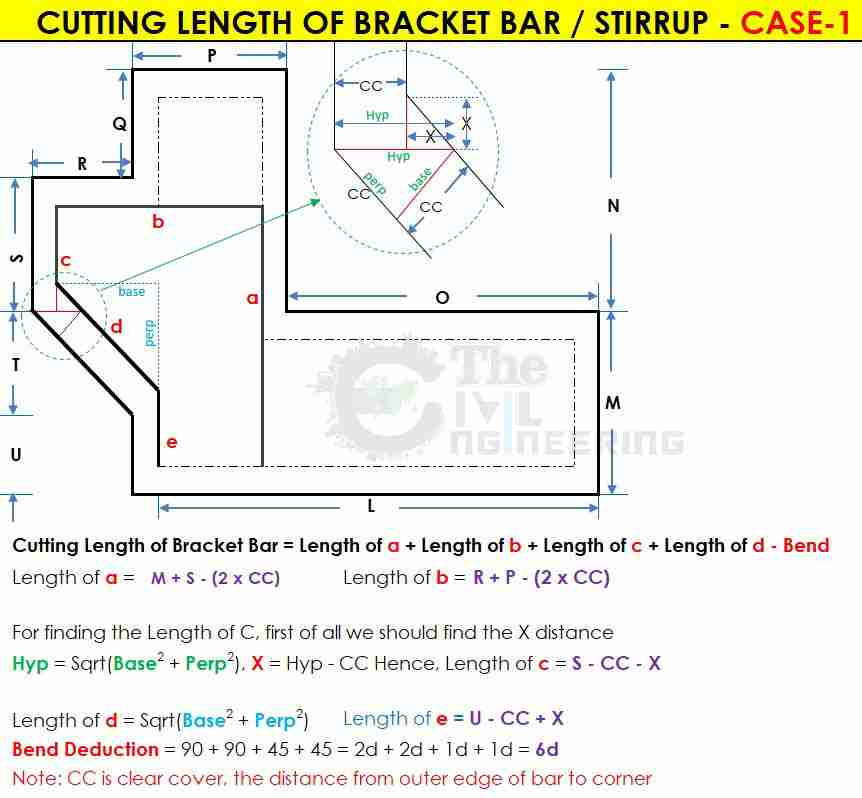## Cutting Length of Bracket Bar Formula – Case 2: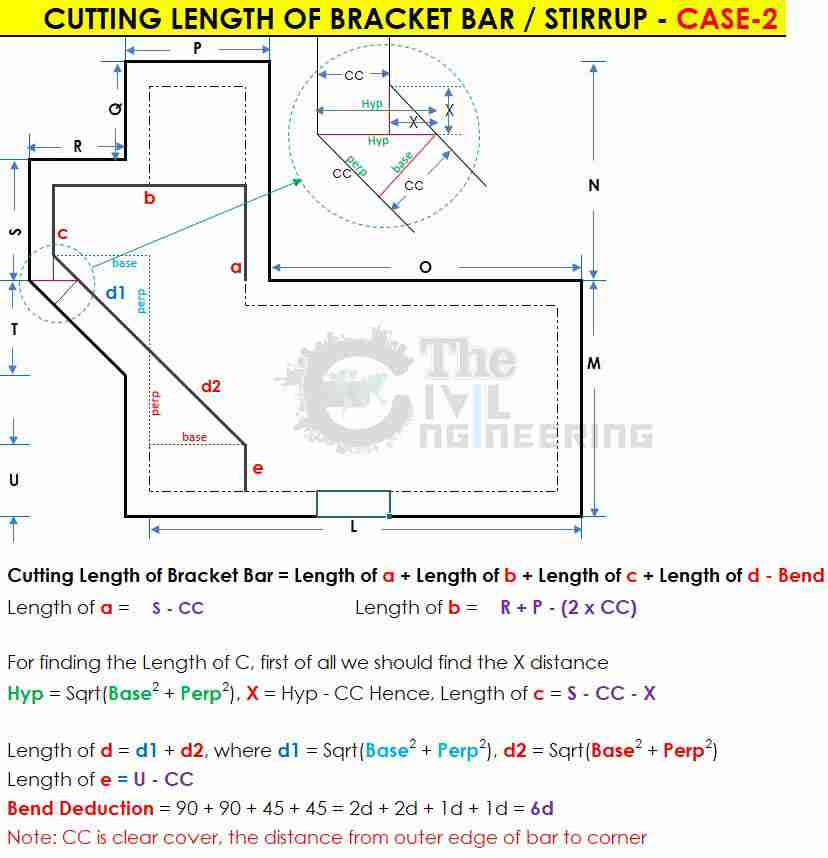## Cutting Length of Four Legged Stirrups Formula: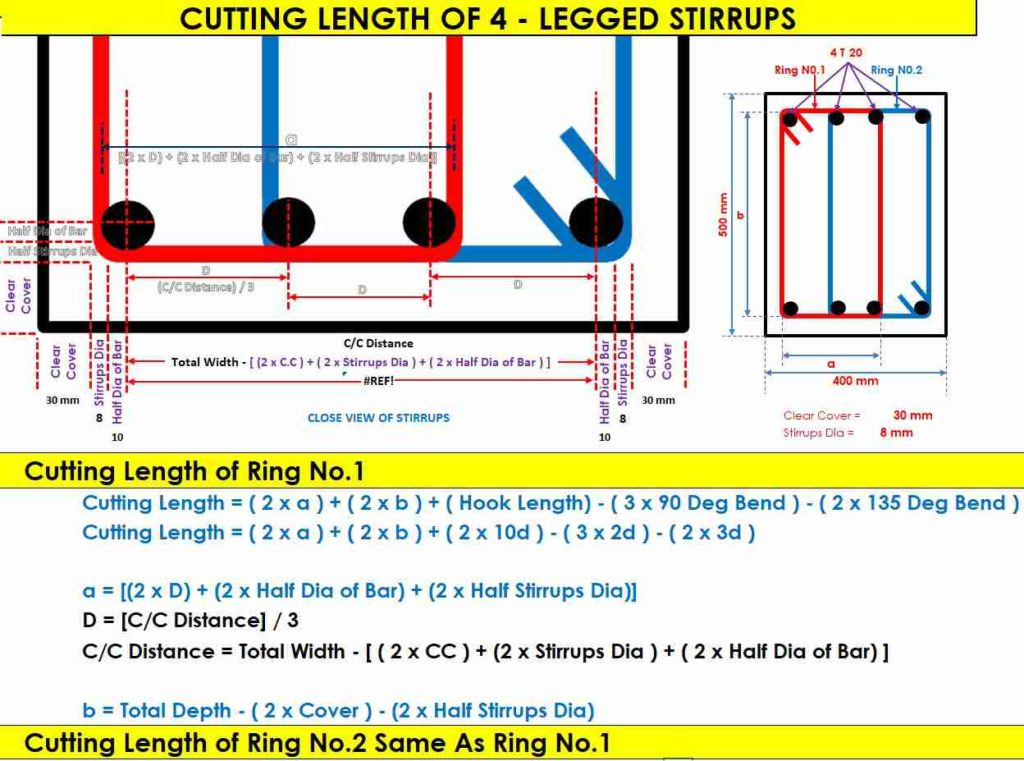## Cutting Length of Six Legged Stirrups Formula: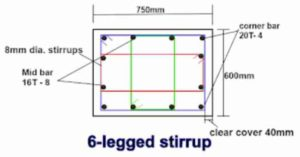For Calculating the Cutting Length of Stirrups, First of all we convert the stirrups in three parts (Stirrups 1, Stirrups 2, Stirrups 3)

Cutting Length of 6-Leggeed Stirrups = Length of Stirrups 1 + Length of Stirrups 2 + Length of Stirrups 3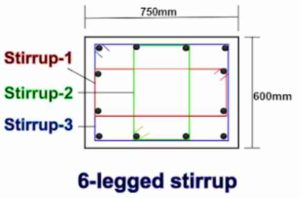### Cutting Length of Stirrups 1: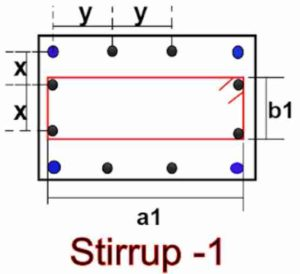#### Cutting Length Formula for Stirrup-1:

= [(2nos × a1) + (2nos. × b1) + (2nos. × hook length) – {(3nos. × 90° bend )+ (2nos. × 135° bend)}]

First, we will calculate the value of  a1 & b1 a1 = [(column width ) – {(2nos. × clear cover) + (2nos.× 1/2 dia. of stirrup-1 bar)}]

b1 = [ x + ( 2nos.× 1/2 dia. of  mid bar.) + ( 2nos. × 1/2 dia of stirrup-1 bar )]
First, we will find out the value of x

x = [column depth – {(2nos.×cover )+( 2nos.× dia. of stirrup-3)+ (2nos.× 1/2 dia. of outer bar)}] ÷ 3
= [(2nos × b2) + (2nos. × a2) + (2nos. × hook length) – { (3nos. × 90° bend )+ (2nos. × 135° bend)}]

First, we will calculate the value of  b2 & a2 b2 = [(column depth ) – {(2nos. × clear cover) + (2nos.× 1/2 dia. of stirrup-2)}]

a2 = [ y + ( 2nos.× 1/2 dia. of  mid bar.) + ( 2nos. × 1/2 dia of stirrup-2 )]
First, we will find out the value of y

y = [column width – {(2nos.×cover )+( 2nos.× dia. of stirrup-3)+ (2nos.× 1/2 dia. of outer bar)}] ÷ 3

= [(2nos.× a3) + (2nos. × b3) + (2nos. × hook length) – {(3nos. × 90° bend )+ (2nos. × 135° bend)}]

First, we will calculate the value of  a3 & b3

a3 = [(column width ) – {(2nos. × clear cover) + (2nos.× 1/2 dia. of stirrup-3)}]

b3 = [(column depth ) – {(2nos. × clear cover) + (2nos.× 1/2 dia. of stirrup-3)}]

## Cutting Length of Mesh Bar Formula: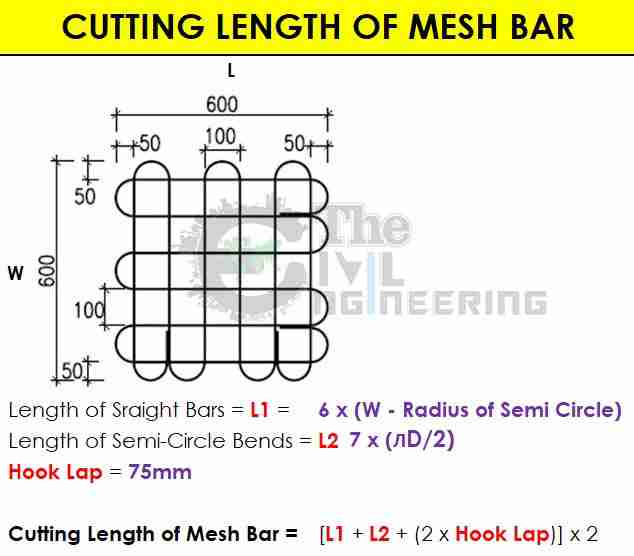## Volume of Steel Reinforcement for Different RCC member:

For Example,

for 10 M3 concrete in Column, considering 2.5% of steel Volume, the column has a 0.25 M3 of steel. Calculate the total weight of steel and steel weight per M3.

Volume of Steel for 10m3 Concrete = 0.25 m3

Wight of Steel Per M3

Concrete = total Volume of steel X Density of Steel (i.e. 7850 Kg/M3)

Weight of steel for 10 M3 concrete = 0.25 X 7850 = 1962.5 Kg.

For 10 m3 Concrete,

the total steel required is 1962.5 Kg. For 1 m3 Concrete, the total Steel required is 196.25 Kg. BBS Sample Excel Sheet

## Rolling Margin of Reinforcement Steel Bar Formula:

Cut reinforcement bar into 3 pieces, each length 1 meter Weight Each piece differently i.e. 10 mm Día Bar, Reinforcement calculation excel

1st one weight 0.617,

2nd one weight 0.62,

3rd one weight 0.625

Now Calculate the rolling margin of Reinforcement bar

## Standard Hook and Bend Length Formula as per ACI 318: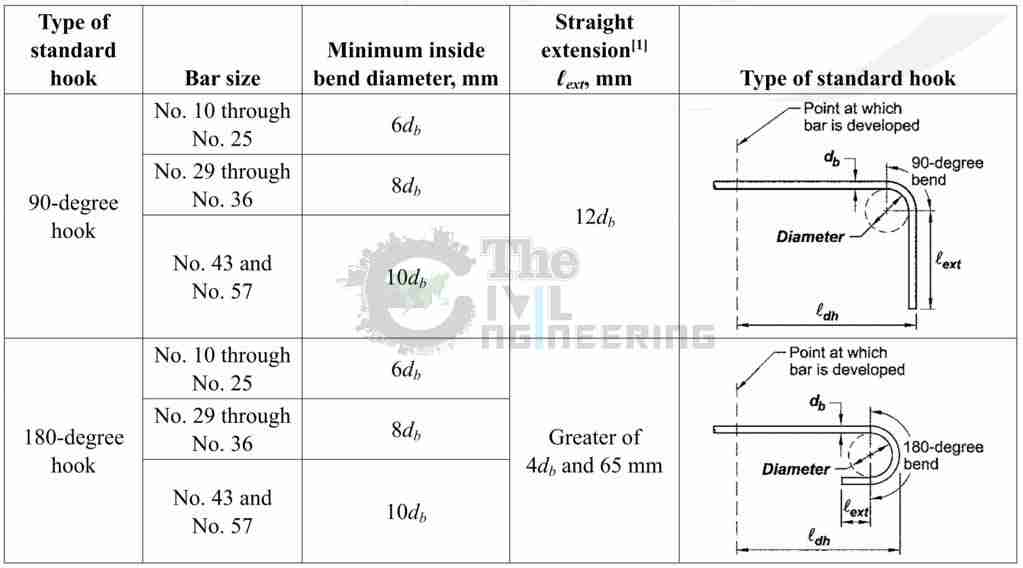## Advantages of Bar Bending Schedule:

• Cutting Length and Bending of Reinforcement can be done.
• BBS avoids wastages of steel reinforcement and also save the project cost
• It provides better estimation of reinforcement steel requirements for each and every structure member. bbs formula
• BBS is very much useful during auditing of reinforcement and provides check or Theft or pilferage. bar bending schedule calculation formula
• It enables easy and fast preparation of Bills of construction work for clients and contractors. Reinforcement calculation excel

## Why Steel is Used as Reinforcement:

• Coefficient of thermal expansion characteristics similar to concrete.
• Good strength for economic design of RCC structures
• Good gripping or good bonding with concrete.
• Good resistance against corrosion for higher durability.
• Good bendability for providing required shape. Rein forcement calculation excel

## Types of Reinforcement Bars:

• Fe-250
• Fe-415
• Fe-500 Basics of Bar Bending Schedule Formulas

## Conclusion:

Full article on What is Bar Bending Schedule | Basics of Bar Bending Schedule Formulas | BBS Formulas | Reinforcement Calculation Excel | How to Make Bar Bending Schedule | Bar Bending Schedule Calculation Formulas. Thank you for the full reading of this article in “The Civil Engineering” platform in English. If you find this post helpful, then help others by sharing it on social media. If any formula of BBS is missing from this article please tell me in comments.

1.Shekar Singam
2.Ashish Yadav